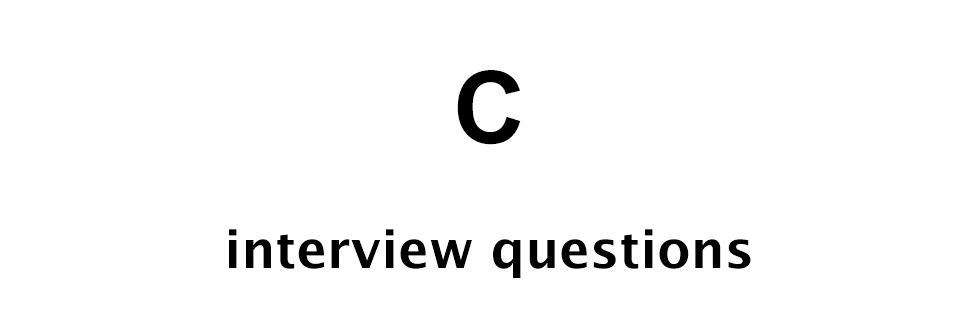# C Interview Questions - Set 3

C Programming Interview Questions## Q1: Print 1 to 1000 without using loop in C programming language

We can write the following C program to print from 1 to 1000 without using loop.

``````#include <stdio.h>

void printNum(int i);

int main() {
printNum(1000);
return 0;
}

void printNum(int lim) {
if (lim > 0) {
printNum(lim - 1);
printf("%d\n", lim);
}
}
``````

In the above code we are using recursion. As long as `lim > 0` inside the `printNum` function we are calling printNum function with a new value of lim which is 1 less than the previous call.

## Q2: What is L-value?

L-value or location value is the expression on the left side of the assignment operator.

Example:

``````int n = 10;
``````

## Q3: What are the types of L-value?

There are two types of L-value.

• Modifiable
• Non-modifiable

Modifiable L-values are the ones that can be assigned any value i.e. modified at a later stage of code execution.

Example:

``````// x is modifiable
int x = 10;

// updating
x = 20;
``````

Non-modifiable L-value are the ones that can't be assigned any new value i.e. can't be modified later.

Example:

``````const float PI = 3.14;
``````

## Q4: What is the use of printf and scanf functions in C?

We use `printf()` function to output result and we use `scanf()` function to take input.

Example:

``````int num;

// take input
scanf("%d", &num);

// show output
printf("%d", num);
``````

## Q5: What is the difference between local and global variable?

Local variable is declared inside a function or a block whereas, global variable is declared outside any function or a block.

Example:

``````#include <stdio.h>

int globalN = 10;

int main() {

int localN = 20;

printf("Global N: %d\n", globalN);
printf("Local N: %d\n", localN);

return 0;
}
``````

## Q6: What is the use of a function in C?

Functions in C helps to break the code into modules and reduces code repetition.

For example, if we have to find the area of a square in our program at 5 different places then we can either write the area code at five different places or we can create a function and call it from those 5 places.

And using the function approach will reduce code repetition and if in future we want to modify the area code then we have to make changes only in that function and not at 5 different places.

## Q7: What is the difference between printf and sprintf in C?

We use `printf` function to output formatted result whereas, we use `sprintf` function to get formatted string which can be used in our code.

## Q8: Write C program to get user data using scanf, format data using sprintf and output result using printf

We can write the following C code to use the three functions.

``````#include <stdio.h>

int main() {

int number;
char *result;

// input
scanf("%d", &number);

// format
sprintf(result, "Entered number is %d\n", number);

// output
printf("%s", result);

return 0;
}
``````

## Q9: What is recursion in C?

When a function calls itself we call it a recursion and the function is called the recursive function.

## Q10: Using recursion print the characters of "Hello World" string

We can write the following code for this.

``````#include <stdio.h>
#include <string.h>

void printChar(char *, int);

int main() {

char *str = "Hello World";
int len = strlen(str);

printChar(str, len - 1);

return 0;
}

void printChar(char *str, int i) {
if (i > -1) {
printChar(str, i - 1);
printf("%c\n", str[i]);
}
}
``````